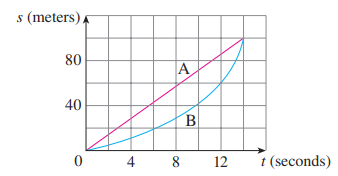×

×

# Solved: Shown are graphs of the position functions of two runners, A and B, who run aISBN: 9781305270336 484

## Solution for problem 12 Chapter 2.7

Single Variable Calculus: Early Transcendentals | 8th Edition

• Textbook Solutions
• 2901 Step-by-step solutions solved by professors and subject experts
• Get 24/7 help from StudySoup virtual teaching assistantsSingle Variable Calculus: Early Transcendentals | 8th Edition

4 5 1 278 Reviews
19
4
Problem 12

Shown are graphs of the position functions of two runners, A and B, who run a 100-meter race and finish in a tie. s (meters) 0 4 8 12 80 40 t (seconds) A B (a) Describe and compare how the runners run the race. (b) At what time is the distance between the runners the greatest? (c) At what time do they have the same velocity?

Step-by-Step Solution:

Step 1 of 3

Shown are graphs of the position functions of two runners, A and B, who run a 100-meter race and finish in a tie.From the given graph it is seen that the runner runs a 100- meter race along a straight line.

So, the velocity of runner A is constant throughout the race.

The runner B follows a non-linear path. Therefore, the velocity of the runner throughout the whole race is not constant.

Step 2 of 3

Step 3 of 3

##### ISBN: 9781305270336

This full solution covers the following key subjects: . This expansive textbook survival guide covers 95 chapters, and 5427 solutions. Since the solution to 12 from 2.7 chapter was answered, more than 229 students have viewed the full step-by-step answer. The answer to “Shown are graphs of the position functions of two runners, A and B, who run a 100-meter race and finish in a tie. s (meters) 0 4 8 12 80 40 t (seconds) A B (a) Describe and compare how the runners run the race. (b) At what time is the distance between the runners the greatest? (c) At what time do they have the same velocity?” is broken down into a number of easy to follow steps, and 67 words. The full step-by-step solution to problem: 12 from chapter: 2.7 was answered by , our top Calculus solution expert on 03/19/18, 03:29PM. This textbook survival guide was created for the textbook: Single Variable Calculus: Early Transcendentals, edition: 8. Single Variable Calculus: Early Transcendentals was written by and is associated to the ISBN: 9781305270336.

Unlock Textbook Solution